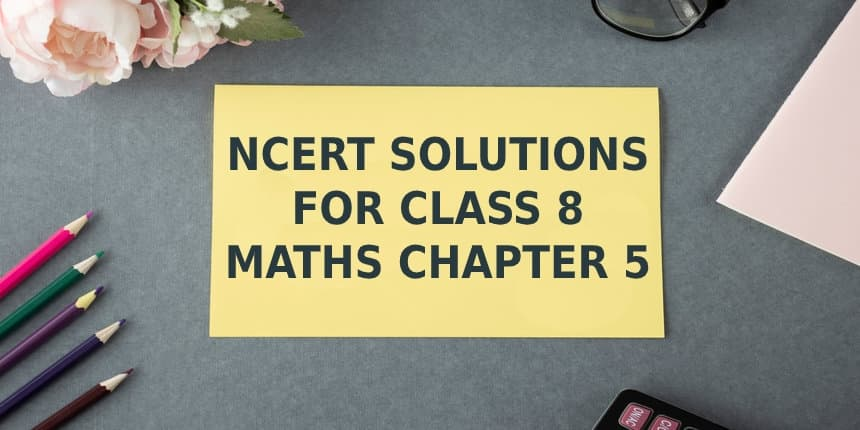# NCERT Solutions For  Class 8 Maths Chapter – 5  Data Handling Exercise – 5.2Q1. A survey was made to find the type of music that type of music that a certain group of young people liked in a city.

Adjoining pie chart shows the finding of this survey. From this pie chart, answer the following:

• Which type of music is liked by the maximum number of people?
• If a cassette company were to make 100% CD
• how many of each type of would they make?

Solution:-

• 10% represents 100 people.

20% represents = ( 100 x 20 ) / 10 = 200

200 people were surveyed.

• Since 40% of the total people surveyed liked night music and no other form of song liked more than that, we can conclude that light music is liked by the maximum number of people
• CD of classical music = ( 10 x 1000)/100 = 100

CD of semi classical music = ( 20 x 1000)/100 = 200

CD of light music = ( 40 x 1000)/100 = 400

CD of folk music = ( 30 x 1000)/100 = 300

Q2. A group of 360 people were asked to vote for their favourite season from the three

Season rainy , winter and summer.

• Which season got the most votes ?
• Find the central angle of each sector.
• Draw a pie chart to show this information

Solution:-

• According to the table given in the question , Winter season got the most votes.
• Central angle of summer season = ( 90 x 360)/360 = 900

Central angle of rainy season = ( 120 x 360)/360 = 1200

Central angle of winter season = ( 150 x 360)/360 = 1500

(iii)

Q3. Draw a pie chart showing the following information. The tables shows the colours preferred by a group of people.

Solution:-

Here , central angle = 3600 Total number  of people = 36

Q4. The adjoining pie chart gives the marks scored in an examination by a student in Hindi, English , Mathematics , Social studies and Science If the total  marks obtained by the students were 540 , answer the following questions.

• In which subject did the student score 105 marks?

( Hint : for 540 marks , the central angle = 3600 So, for 105 marks, what is the central angles ? )

• How many more marks were obtained by the student in Mathematics than in Hindi?
• Examine whether the sum of the marks obtained in Social Science and Mathematics is more than that in Science and Hindi. ( Hint : Just study the central angles).

Solution:-

• The student scored 105 marks in Hindi.
• Marks obtained in Mathematics = 135 Marks obtained in Hindi = 105 Difference = 135 – 105 = 30

Thus, 30 more marks were obtained by the student in Mathematics than in Hindi.

• The sum of marks in Social Science and Mathematics = 97.5 + 135 = 232 .5 The sum of marks in science and Hindi = 120 + 105 = 225

The sum of the marks in Social Science and Mathematics is more than that in Science and Hindi.

Q5. The number of students in a hostel , speaking different languages is given below . Display the data in a pie chart.

Solution:-

NCERT Solutions for class 7 English chapter 10the story of cricket

NCERT Solutions For  Class 8 Maths Chapter – 5  Data Handling Exercise – 5.2 Q1. A survey was made to find…